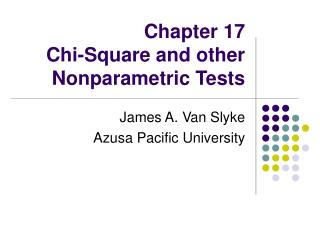DownloadDownload PresentationChapter 17 Chi-Square and other Nonparametric Tests

Chapter 17 Chi-Square and other Nonparametric Tests

Télécharger la présentationChapter 17 Chi-Square and other Nonparametric Tests

- - - - - - - - - - - - - - - - - - - - - - - - - - - E N D - - - - - - - - - - - - - - - - - - - - - - - - - - -
Presentation Transcript

1. Chapter 17 Chi-Square and other Nonparametric Tests James A. Van Slyke Azusa Pacific University

2. Distinctions between Parametric and Nonparametric Tests • Parametric tests (e. g. t, z) depend substantially on population characteristics • Nonparametric tests depend minimally on population parameters • Fewer requirements • Often referred to as distribution free tests • Advantages for parametric tests • Generally more powerful and versatile • Generally robust to violations of the test assumptions (sampling differences)

3. Chi-Square (χ2) Single Variable Experiments • Often used with nominal data • Allows one to test if the observed results differ significantly from the results expected if H0 were true

4. Chi-Square (χ2) Single Variable Experiments • Computational formula

5. Chi-Square (χ2) Single Variable Experiments • Evaluation of Chi-Square obtained • Family of Curves • Vary with degrees of freedom • k-1 degrees of freedom where k equals the number of groups or categories • The larger the discrepancy between the observed and expected results the larger the value of chi-square, the more unreasonable that H0 is accepted

6. Chi-Square: Test of independence between two variables • Used to determine whether two variables are related • Contingency table • This is a two-way table showing the contingency between two variables • The variables have been classified into mutually exclusive categories and the cell entries are frequencies

7. Chi-Square: Test of independence between two variables • Null hypothesis states that the observed frequencies are due to random sampling from a population • This population has proportions in each category of one variable that are the same for each category of the over variable • Alternative hypothesis is that these proportions are different

8. Chi-Square: Test of independence between two variables • Calculation of chi-squared for contingency tables • fe can be found by multiplying the marginals (i.e. row and column totals lying outside the table) and dividing by N • Sum (fo – fe)2/fe for each cell

9. Chi-Square: Test of independence between two variables • Evaluation of chi-square • df = number of fo scores that are free to vary • While at the same time keeping the column and row marginals the same • Equation • df = (r – 1)(c – 1) • Where r = number of rows in the contingency table • c = number of columns in the contingency table